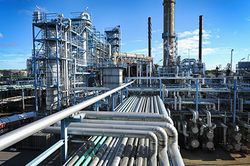# Documentation:CHBE Exam Wiki/Midterm Exam 2 2016W/MT2 Question 3

CHBE 241
Exam resources wikiChemical and Biological Engineering
Welcome to the CHBE Exam Resources Wiki!

This wiki is intended to host past exams

with fully worked-out hints and solutions
Past Exams
Final Exam 2016W
Midterm Exam 1 2016W
Midterm Exam 2 2016W
Problem Sets
Module 1 - Process Basics
Module 2 - Reactors
Module 3 - Separations 1
Module 4 - Separations 2
Module 5 - Non-reactive Energy Balances
Module 6 - Reactive Energy Balances

Catalytic converters are used in cars to oxidize emission compounds in order to make them less toxic. A similar process may be used in a chemical plant to clean up emissions. A waste gas contains 0.5 mol% C3H8 (propane), 8.5 mol% CO2, 4 mol% O2, 10 mol% H2O and the remainder inert nitrogen (N2). In order to try to remove the propane, it undergoes complete combustion with a catalyst and can be modeled as a reversible reaction. This reaction is undertaken at 1 atm.

## Question 3a [5 points]

Is there excess oxygen, if so, what is the % excess of oxygen?

## Question 3b [15 points]

Write out a formula for the equilibrium constant (Ke) in terms of the pressure (P), reaction extent (E) and molar feed (n°C3H8, n°CO2, n°O2, n°H2O, n°N2) of each compound. Simplify the expression algebraically as much as possible. Note that you should not need to perform any calculations for this.

## Question 3c [15 points]

A conversion of 95% of propane from the combustion reaction is achieved. Following this, the remaining propane is removed using an adsorption bed at the same pressure containing 1 tonne activated carbon. Laboratory experiments were undertaken to model the adsorption of propane and gave the results found below, given this how many kilograms of propane can this bed remove?

$X^{*}(g_{C_{3}H_{8}}/100g_{\text{activated carbon}})=K'\cdot p_{C_{3}H_{8}}$where $K'=67g_{C_{3}H_{8}}/(kPa*100g_{\text{activated carbon}})$Script error: The function "navbox" does not exist.